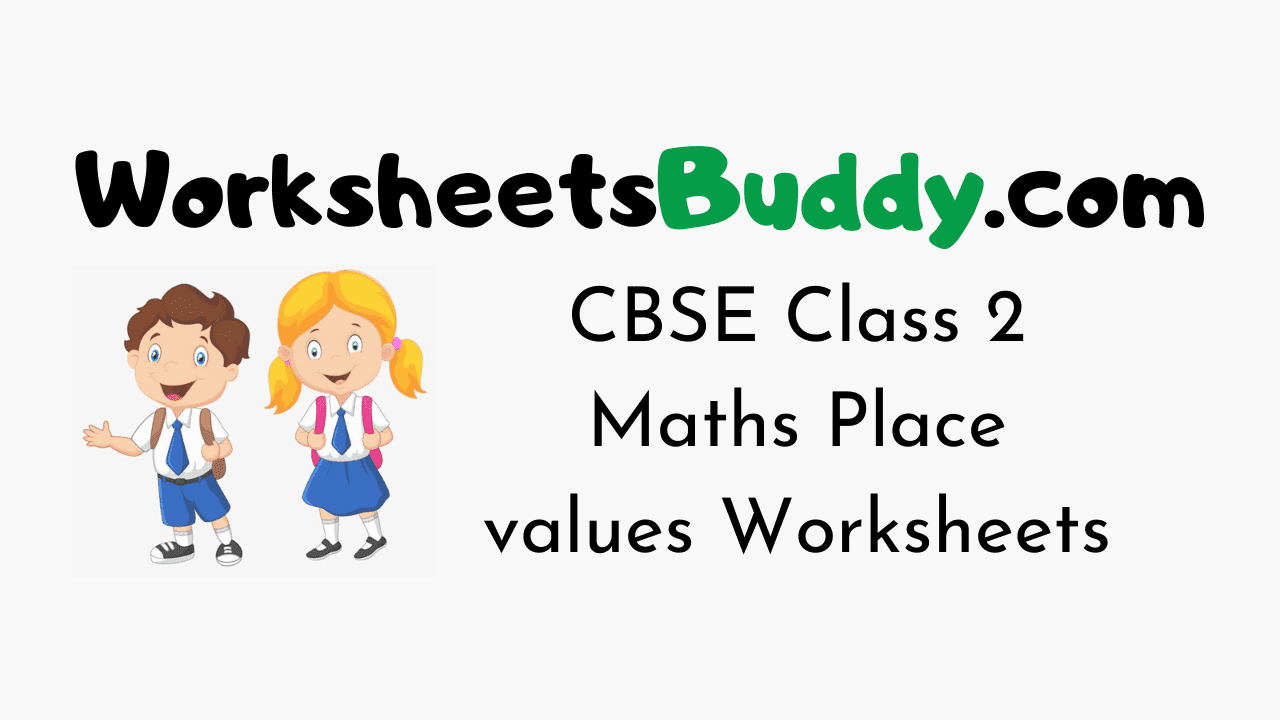# CBSE Class 2 Maths Place values Worksheets

CBSE Class 2 Maths Place values Worksheets – Worksheets have become an integral part and are the most engaging study materials for kids these days. The Standard 2 Maths Place values Worksheets will help your younger one to practice what they have learned in class. Place values Worksheets for Grade 2 will develop a curiosity in your kid to learn.

Maths Worksheets for Class 2 are a perfect combination of fun and learning. Find the most interesting and relevant puzzle activities for your kids here. In the Mathematics Grade 2 Place values Worksheets Puzzles will be in the form of crosswords, true or false, pictures, mazes, fill-ups, MCQs, objectives, questions and answers, and so on.

Mathematics Worksheets for Class 2 on Place values will help your kid remember what he/ she learned in school for a long time. Your Grade 2 kid will love to solve these engaging exercises and interesting collections of Puzzles.

### CBSE Class 2 Maths Place values Worksheets

1. Fill in the blanks: 7 Tens + 7 Ones = ______

2. Write the following in expanded form: 71

3. Compare using (<, >, =): 4 Tens 6 Ones _____ 5 Tens 6 Ones
A) <
B) >
C) =

4. Fill in the blanks: ____ Tens + _____ Ones = 10

5. Fill in the blanks: 5 Tens + 4 Ones = ______

6. Compare using (<, >, =): 3 Tens 1 Ones _____ 4 Tens 3 Ones
A) <
B) >
C) =

7. Write the following in expanded form: 55

8. Write the following in expanded form: 48

9. Fill in the blanks: 2 Tens + 3 Ones = ______

10. Fill in the blanks: ____ Tens + _____ Ones = 16

11. Fill in the blanks: 3 Tens + 6 Ones = ______

12. Fill in the blanks: ____ Tens + _____ Ones = 80

13. Fill in the blanks: ____ Tens + _____ Ones = 99

14. Fill in the blanks: ____ Tens + _____ Ones = 37

15. Write the following in expanded form: 26

16. Compare using (<, >, =): 1 Tens 0 Ones _____ 4 Tens 3 Ones
A) <
B) >
C) =

17. Fill in the blanks: ____ Tens + _____ Ones = 28

18. Fill in the blanks: 9 Tens + 7 Ones = ______

19. Write the following in expanded form: 69

20. Fill in the blanks: ____ Tens + _____ Ones = 11

### CBSE Class 2 Maths Worksheet Answers

1. 77
2. 70 + 1
3. Option A
4. 1, 0
5. 54
6. Option A
7. 50 + 5
8. 40 + 8
9. 23
10. 1, 6
11. 36
12. 8, 0
13. 9, 9
14. 3, 7
15. 20 + 6
16. Option A
17. 2, 8
18. 97
19. 60 + 9
20. 1, 1### FAQs on Maths Place values Class 2 Worksheets

1. How does a child learn Mathematics Place values at Class 2 Level?

A child can learn Maths Place values at Class 2 Level through the worksheets prevailing on our page.

2. What are the topics in Maths Class 2?

Mathematics Class 2 will have topics like Place values etc.

3. Where can I get Mathematics Worksheets for Class 2 Place values for free?

You can get Maths Place values Worksheets Class 2 free of cost from our Worksheetsbuddy.com page. You can use them for your kid to get practice.

4. Are the CBSE Class 2 Place values Worksheets over here printable?

Yes, the CBSE Class 2 Place values Worksheets here are printable and you can download them easily.

Conclusion

We Hope the information prevailing on our page regarding CBSE Class 2 Mathematics Place values Worksheets has been useful to you. For more info feel free to reach us and we will assist you at the easiest possible. Keep in touch with our site for more information on Class Wise Worksheets for various subjects.